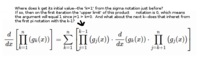# Mathematical Induction

#### MarkFL

##### Super Moderator
Staff member
This is how I would work the problem:

The product rule for a composite function that is the product of two functions is well-known and will be the basis for working this problem (and accepted without proof):

$$\displaystyle \frac{d}{dx}\left(g_1(x)\cdot g_2(x) \right)=g_1'(x)\cdot g_2(x)+g_1(x)\cdot g_2'(x)$$

Using this rule, let's look at:

$$\displaystyle \frac{d}{dx}\left(g_1(x)\cdot g_2(x)\cdot g_3(x) \right)$$

Now, let's associate two of the functions together, it doesn't matter which two, so let's use the first two:

$$\displaystyle \frac{d}{dx}\left(\left(g_1(x)\cdot g_2(x) \right)\cdot g_3(x) \right)$$

Now, using the product rule above, we may state:

$$\displaystyle \frac{d}{dx}\left(\left(g_1(x)\cdot g_2(x) \right)\cdot g_3(x) \right)=\frac{d}{dx}\left(g_1(x)\cdot g_2(x) \right)\cdot g_3(x)+\left(g_1(x)\cdot g_2(x) \right)\cdot g_3'(x)$$

Using the product rule again, we find:

$$\displaystyle \frac{d}{dx}\left(\left(g_1(x)\cdot g_2(x) \right)\cdot g_3(x) \right)=\left(g_1'(x)\cdot g_2(x)+g_1(x)\cdot g_2'(x) \right)\cdot g_3(x)+\left(g_1(x)\cdot g_2(x) \right)\cdot g_3'(x)$$

And distributing, we find:

$$\displaystyle \frac{d}{dx}\left(\left(g_1(x)\cdot g_2(x) \right)\cdot g_3(x) \right)=g_1'(x)\cdot g_2(x)\cdot g_3(x)+g_1(x)\cdot g_2'(x)\cdot g_3(x)+g_1(x)\cdot g_2(x)\cdot g_3'(x)$$

Now, this is enough to suggest the pattern (our induction hypothesis $$P_n$$):

$$\displaystyle \frac{d}{dx}\left[\prod_{k=1}^n\left(g_k(x) \right) \right]=\sum_{k=1}^n\left[\prod_{j=1}^{k-1}\left(g_j(x) \right)\cdot\frac{d}{dx}\left(g_k(x) \right)\cdot\prod_{j=k+1}^n\left(g_j(x) \right) \right]$$

Next, consider:

$$\displaystyle \frac{d}{dx}\left[\prod_{k=1}^n\left(g_k(x) \right)\cdot g_{n+1}(x) \right]$$

Using the product rule, and incorporating the new factor into the product. we may state:

$$\displaystyle \frac{d}{dx}\left[\prod_{k=1}^{n+1}\left(g_k(x) \right) \right]=\frac{d}{dx}\left[\prod_{k=1}^n\left(g_k(x) \right) \right]\cdot g_{n+1}(x)+\prod_{k=1}^n\left(g_k(x) \right)\cdot g_{n+1}'(x)$$

Using our induction hypothesis, this becomes:

$$\displaystyle \frac{d}{dx}\left[\prod_{k=1}^{n+1}\left(g_k(x) \right) \right]=\sum_{k=1}^n\left[\prod_{j=1}^{k-1}\left(g_j(x) \right)\cdot\frac{d}{dx}\left(g_k(x) \right)\cdot\prod_{j=k+1}^n\left(g_j(x) \right) \right]\cdot g_{n+1}(x)+\prod_{k=1}^n\left(g_k(x) \right)\cdot g_{n+1}'(x)$$

Now, incorporating the factor at the end of the first term on the right, we have:

$$\displaystyle \frac{d}{dx}\left[\prod_{k=1}^{n+1}\left(g_k(x) \right) \right]=\sum_{k=1}^n\left[\prod_{j=1}^{k-1}\left(g_j(x) \right)\cdot\frac{d}{dx}\left(g_k(x) \right)\cdot\prod_{j=k+1}^{n+1}\left(g_j(x) \right) \right]+\prod_{k=1}^n\left(g_k(x) \right)\cdot g_{n+1}'(x)$$

And finally incorporating the second term on the right within the first summation term, we have:

$$\displaystyle \frac{d}{dx}\left[\prod_{k=1}^{n+1}\left(g_k(x) \right) \right]=\sum_{k=1}^{n+1}\left[\prod_{j=1}^{k-1}\left(g_j(x) \right)\cdot\frac{d}{dx}\left(g_k(x) \right)\cdot\prod_{j=k+1}^{n+1}\left(g_j(x) \right) \right]$$

We have derived $$P_{n+1}$$ from $$P_n$$, thereby completing the proof by induction.

•topsquark

#### cambium0

##### New member
First, thank you very much for your detailed reply.

I am not familiar with the workings of the sigma and pi operators beyond the most basic cases, and I'm interpreting them as a programmer would interpret nested loops. So I get confused at your induction hypothesis (Pn) line. Beginning at the First sigma of the line that follows ("our induction hypothesis, Pn"), you have a summation of products of functions through n, one addend for each factor. Good. Let's say n is 3. Now we enter the square brackets, and 1?)apply k==1 from the sigma notation to the upper limit expression of k-1 of the first pi notation, obtaining k==0? And the product operator index j is set equal to 1. I looked it up on the internet and found that when the upper limit is less than the index, the value of that factor is 1 (as close to nothing as possible while preserving the other terms). Now if the k in 'k-1' does not get it's value from the k in the sigma notation, then where does it get its initial value? So I assume I'm right, which means that block is not working like you think it is. So if you don't mind, i need to know what the scope of the k and j variables are if they are different than what it appears. At any rate, if you write out the addends according to your induction hypothesis formulation, i get

n=1|| g1(x) +
n=2|| (g1(x) * d/dxg1(x)) * ( g2(x) * g3(x)) +
n=3|| (g1(x)* d/dxg1(x)) * (g3(x)

#### MarkFL

##### Super Moderator
Staff member
Let's examine the induction hypothesis:

$$\displaystyle \frac{d}{dx}\left[\prod_{k=1}^n\left(g_k(x) \right) \right]=\sum_{k=1}^n\left[\prod_{j=1}^{k-1}\left(g_j(x) \right)\cdot\frac{d}{dx}\left(g_k(x) \right)\cdot\prod_{j=k+1}^n\left(g_j(x) \right) \right]$$

Now, let $$n=1$$:

$$\displaystyle \frac{d}{dx}\left[\prod_{k=1}^1\left(g_k(x) \right) \right]=\sum_{k=1}^1\left[\prod_{j=1}^{k-1}\left(g_j(x) \right)\cdot\frac{d}{dx}\left(g_k(x) \right)\cdot\prod_{j=k+1}^1\left(g_j(x) \right) \right]$$

This reduces to:

$$\displaystyle \frac{d}{dx}\left(g_1(x)\right)=\frac{d}{dx}\left(g_1(x)\right)\quad\checkmark$$

Let $$n=2$$:

$$\displaystyle \frac{d}{dx}\left[\prod_{k=1}^2\left(g_k(x) \right)\right]=\sum_{k=1}^2\left[\prod_{j=1}^{k-1}\left(g_j(x) \right)\cdot\frac{d}{dx}\left(g_k(x) \right)\cdot\prod_{j=k+1}^2\left(g_j(x) \right) \right]$$

This reduces to:

$$\displaystyle \frac{d}{dx}\left(g_1(x)\cdot g_2(x)\right)=\frac{d}{dx}\left(g_1(x)\right)\cdot g_2(x)+g_1(x)\cdot\frac{d}{dx}\left(g_1(x)\right)\quad\checkmark$$

By the way, the proof I presented here is one I have posted as tutorials on 2 other math help sites (that's why I presented the complete proof), and no one so far has posted any issues with the formulas not working or being correct. That is no guarantee of course that there isn't an error, but I felt it worth mentioning.

I'm not entirely certain what you mean when you said:

"Now if the k in 'k-1' does not get it's value from the k in the sigma notation, then where does it get its initial value?"

Can you clarify?

•topsquark

#### cambium0

##### New member
Let's examine the induction hypothesis:

$$\displaystyle \frac{d}{dx}\left[\prod_{k=1}^n\left(g_k(x) \right) \right]=\sum_{k=1}^n\left[\prod_{j=1}^{k-1}\left(g_j(x) \right)\cdot\frac{d}{dx}\left(g_k(x) \right)\cdot\prod_{j=k+1}^n\left(g_j(x) \right) \right]$$

Now, let $$n=1$$:

$$\displaystyle \frac{d}{dx}\left[\prod_{k=1}^1\left(g_k(x) \right) \right]=\sum_{k=1}^1\left[\prod_{j=1}^{k-1}\left(g_j(x) \right)\cdot\frac{d}{dx}\left(g_k(x) \right)\cdot\prod_{j=k+1}^1\left(g_j(x) \right) \right]$$

This reduces to:

$$\displaystyle \frac{d}{dx}\left(g_1(x)\right)=\frac{d}{dx}\left(g_1(x)\right)\quad\checkmark$$

Let $$n=2$$:

$$\displaystyle \frac{d}{dx}\left[\prod_{k=1}^2\left(g_k(x) \right)\right]=\sum_{k=1}^2\left[\prod_{j=1}^{k-1}\left(g_j(x) \right)\cdot\frac{d}{dx}\left(g_k(x) \right)\cdot\prod_{j=k+1}^2\left(g_j(x) \right) \right]$$

This reduces to:

$$\displaystyle \frac{d}{dx}\left(g_1(x)\cdot g_2(x)\right)=\frac{d}{dx}\left(g_1(x)\right)\cdot g_2(x)+g_1(x)\cdot\frac{d}{dx}\left(g_1(x)\right)\quad\checkmark$$

By the way, the proof I presented here is one I have posted as tutorials on 2 other math help sites (that's why I presented the complete proof), and no one so far has posted any issues with the formulas not working or being correct. That is no guarantee of course that there isn't an error, but I felt it worth mentioning.

I'm not entirely certain what you mean when you said:

"Now if the k in 'k-1' does not get it's value from the k in the sigma notation, then where does it get its initial value?"

Can you clarify?So n=1: k=1, going to first pi notation, k-1 == 0? if so, you have j=1, k=0, and the internet tells me that means no matter what the argument of the pi notation is, it's equal to 1. If I could understand the way these variables work I might get a handle on this.

#### MarkFL

##### Super Moderator
Staff member
Yes, in both pi and sigma notation if the upper index is less than the lower index, then the entire product/sum represented within that indexing essentially evaluates to 1.